# Turbine

Turbine for boosted engines

•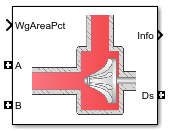Libraries:
Powertrain Blockset / Propulsion / Combustion Engine Components / Boost

## Description

The Turbine block uses the conservation of mass and energy to calculate mass and heat flow rates for turbines with either fixed or variable geometry. You can configure the block with a wastegate valve to bypass the turbine. The block uses two-way ports to connect to the inlet and outlet control volumes and the drive shaft. You can specify the lookup tables to calculate the mass flow rate and turbine efficiency. Typically, turbine manufacturers provide the mass flow rate and efficiency tables as a function of corrected speed and pressure ratio. The block does not support reverse mass flow.

If you have Model-Based Calibration Toolbox™, click Calibrate Performance Maps to virtually calibrate the mass flow rate and turbine efficiency lookup tables using measured data.

The mass flows from the inlet control volume to outlet control volume.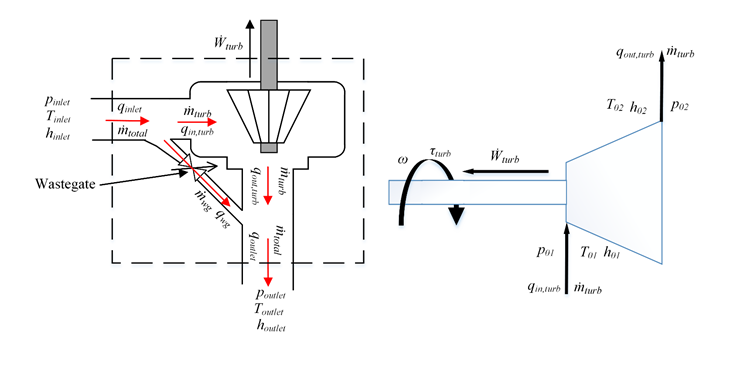The Turbine block implements equations to model the performance, wastegate flow, and combined flow.

### Virtual Calibration

If you have Model-Based Calibration Toolbox, click Calibrate Performance Maps to virtually calibrate the corrected mass flow rate and turbine efficiency lookup tables using measured data. The dialog box steps through these tasks.

Description

Import turbine data

Import this turbine data from a file. For more information, see Using Data (Model-Based Calibration Toolbox).

Turbine type

Data

`Fixed geometry`

• Corrected mass flow rate, MassFlwRate, in kg/s

• Pressure ratio, PrsRatio, dimensionless

• Efficiency, Eff, dimensionless

The speed, corrected mass flow rate, pressure ratio, and efficiency are in the 2nd-5th columns of the data file, respectively. The first and second rows of the data file provide the variable names and units. For example, use this format.

 Name: Spd MassFlwRate PrsRatio Eff Unit: rad/s kg/s Data: `8373.3` `0.02` `1.21` `0.44` ... ... ... ...

```Variable geometry```

• Corrected mass flow rate, MassFlwRate, kg/s

• Rack position, RackPos, dimensionless

• Pressure ratio, PrsRatio, dimensionless

• Efficiency, Eff, dimensionless

Include data for several test points at each rack position operating point.

The speed, corrected mass flow rate, rack position, pressure ratio, and efficiency are in the 2nd-6th columns of the data file, respectively. The first and second rows of the data file provide the variable names and units. For example, use this format.

 Name: Spd MassFlwRate RackPos PrsRatio Eff Unit: rad/s kg/s Data: `8373.3` `0.02` `1` `1.21` `0.44` ... ... ... ...

Model-Based Calibration Toolbox limits the speed and pressure ratio breakpoint values to the maximum values in the file.

To filter or edit the data, select . The Model-Based Calibration Toolbox Data Editor opens.

Generate response models

Model-Based Calibration Toolbox fits the imported data and generates response models.

Turbine type

Description

`Fixed geometry`

Data

Response Model

Corrected mass flow rate

Square root turbine flow model described in Modeling and Control of Engines and Drivelines2

Efficiency

Blade speed ratio (BSR) model described in Modeling and Control of Engines and Drivelines2

`Variable geometry`

Model-Based Calibration Toolbox uses a point-by-point test plan to fit the data. For each rack position, the block uses these response models to fit the corrected mass flow rate and efficiency data.

Data

Response Model

Corrected mass flow rate

Square root turbine flow model described in Modeling and Control of Engines and Drivelines2

Efficiency

Blade speed ratio (BSR) model described in Modeling and Control of Engines and Drivelines2

To assess or adjust the response model fit, select . The Model-Based Calibration Toolbox Model Browser opens. For more information, see Model Assessment (Model-Based Calibration Toolbox).

Generate calibration

Model-Based Calibration Toolbox calibrates the response model and generates calibrated tables.

Turbine type

Description

`Fixed geometry`

Model-Based Calibration Toolbox uses the response models for the corrected mass flow rate and efficiency tables.

`Variable geometry`

Model-Based Calibration Toolbox fills the corrected mass flow rate and efficiency tables for each rack position. Model-Based Calibration Toolbox then combines the rack position-dependent tables into 3D lookup tables for corrected mass flow rate and efficiency.

To assess or adjust the calibration, select . The Model-Based Calibration Toolbox CAGE Browser opens. For more information, see Calibration Lookup Tables (Model-Based Calibration Toolbox).

Update block parameters

Update these corrected mass flow rate and efficiency parameters with the calibration.

Turbine type

Parameters

`Fixed geometry`
• Corrected mass flow rate table, mdot_corrfx_tbl

• Efficiency table, eta_turbfx_tbl

• Corrected speed breakpoints, w_corrfx_bpts1

• Pressure ratio breakpoints, Pr_fx_bpts2

```Variable geometry```

• Corrected mass flow rate table, mdot_corrvr_tbl

• Efficiency table, eta_turbvr_tbl

• Corrected speed breakpoints, w_corrvr_bpts2

• Pressure ratio breakpoints, Pr_vr_bpts2

• Rack breakpoints, L_rack_bpts3

### Thermodynamics

The block uses these equations to model the thermodynamics.

CalculationEquations
Forward mass flow

${\stackrel{˙}{m}}_{turb}>0$

${p}_{01}={p}_{inlet}$

${p}_{02}={p}_{outlet}$

${T}_{01}={T}_{inlet}$

${h}_{01}={h}_{inlet}$

First law of thermodynamics

${\stackrel{˙}{W}}_{turb}={\stackrel{˙}{m}}_{turb}{c}_{p}\left({T}_{01}-{T}_{02}\right)$

Isentropic efficiency

${\eta }_{turb}=\frac{{h}_{01}-{h}_{02}}{{h}_{01}-{h}_{02\text{s}}}=\frac{{T}_{01}-{T}_{02}}{{T}_{01}-{T}_{02\text{s}}}$

Isentropic outlet temperature, assuming ideal gas, and constant specific heats

${T}_{02s}={T}_{01}{\left(\frac{{p}_{02}}{{p}_{01}}\right)}^{\frac{\gamma -1}{\gamma }}$

Specific heat ratio

$\gamma =\frac{{c}_{p}}{{c}_{p}-R}$

Outlet temperature

${T}_{02}={T}_{01}+{\eta }_{turb}{T}_{01}\left\{1-{\left(\frac{{p}_{02}}{{p}_{01}}\right)}^{\frac{\gamma -1}{\gamma }}\right\}$

Heat flows

${q}_{in,turb}={\stackrel{˙}{m}}_{turb}{c}_{p}{T}_{01}$

${q}_{out,turb}={\stackrel{˙}{m}}_{turb}{c}_{p}{T}_{02}$

Drive shaft torque

${\tau }_{turb}=\frac{{\stackrel{˙}{W}}_{turb}}{\omega }$

The equations use these variables.

 ${p}_{\text{inlet}}$,${p}_{01}$ Inlet control volume total pressure ${T}_{inlet}$, ${T}_{01}$ Inlet control volume total temperature ${h}_{inlet}$, ${h}_{01}$ Inlet control volume total specific enthalpy ${p}_{outlet}$, ${p}_{02}$ Outlet control volume total pressure ${T}_{outlet}$ Outlet control volume total temperature ${h}_{outlet}$ Outlet control volume total specific enthalpy ${\stackrel{˙}{W}}_{turb}$ Drive shaft power ${T}_{02}$ Temperature exiting the turbine ${h}_{02}$ Outlet total specific enthalpy ${\stackrel{˙}{m}}_{turb}$ Turbine mass flow rate ${q}_{in,turb}$ Turbine inlet heat flow rate ${q}_{out,turb}$ Turbine outlet heat flow rate ${\eta }_{turb}$ Turbine isentropic efficiency ${T}_{02s}$ Isentropic outlet total temperature ${h}_{02s}$ Isentropic outlet total specific enthalpy $R$ Ideal gas constant ${c}_{p}$ Specific heat at constant pressure $\gamma$ Specific heat ratio ${\tau }_{turb}$ Drive shaft torque

### Performance Lookup Tables

The block implements lookup tables based on these equations.

CalculationEquation

Corrected mass flow rate

${\stackrel{˙}{m}}_{corr}={\stackrel{˙}{m}}_{turb}\frac{\sqrt{{T}_{01}/{T}_{ref}}}{{p}_{01}/{p}_{ref}}$

Corrected speed

${\omega }_{corr}=\frac{\omega }{\sqrt{{T}_{01}/{T}_{ref}}}$

Pressure expansion ratio

${p}_{r}=\frac{{p}_{01}}{{p}_{02}}$
Efficiency lookup tableFixed geometry (3-D table)${\eta }_{turbfx,tbl}=f\left({\omega }_{corr},{p}_{r}\right)$
Variable geometry (3-D table)${\eta }_{turbvr,tbl}=f\left({\omega }_{corr},{p}_{r},{L}_{rack}\right)$
Corrected mass flow lookup tableFixed geometry (3-D table)${\stackrel{˙}{m}}_{corrfx,tbl}=f\left({\omega }_{corr},{p}_{r}\right)$
Variable geometry (3-D table)${\stackrel{˙}{m}}_{corrvr,tbl}=f\left({\omega }_{corr},{p}_{r},{L}_{rack}\right)$

The equations use these variables.

 ${p}_{01}$ Inlet control volume total pressure ${p}_{r}$ Pressure expansion ratio ${p}_{02}$ Outlet control volume total pressure ${P}_{ref}$ Lookup table reference pressure ${T}_{01}$ Inlet control volume total temperature ${T}_{ref}$ Lookup table reference temperature ${\stackrel{˙}{m}}_{turb}$ Turbine mass flow rate $\omega$ Drive shaft speed ${\omega }_{corr}$ Corrected drive shaft speed ${L}_{rack}$ Variable geometry turbine rack position ${\eta }_{turbfx,tbl}$ Efficiency 3-D lookup table for fixed geometry ${\stackrel{˙}{m}}_{corrfx,tbl}$ Corrected mass flow rate 3-D lookup table for fixed geometry ${\eta }_{turbvr,tbl}$ Efficiency 3-D lookup table for variable geometry ${\stackrel{˙}{m}}_{corrvr,tbl}$ Corrected mass flow rate 3-D lookup table for variable geometry

### Wastegate

To calculate the wastegate heat and mass flow rates, the Turbine block uses a Flow Restriction block. The Flow Restriction block uses the wastegate flow area.

`${A}_{wg}={A}_{wgpctcmd}\frac{{A}_{wgopen}}{100}$`

The equation uses these variables.

 ${A}_{wgpctcmd}$ Wastegate valve area percent command ${A}_{wg}$ Wastegate valve area ${A}_{wgopen}$ Wastegate valve area when fully open

### Combined Flow

To represent flow through the wastegate valve and turbine, the block uses these equations.

CalculationEquations

Blocks not configured with a wastegate valve

${\stackrel{˙}{m}}_{wg}={q}_{wg}=0$

Total mass flow rate

${\stackrel{˙}{m}}_{total}={\stackrel{˙}{m}}_{turb}+{\stackrel{˙}{m}}_{wg}$

Total heat flow rate

${q}_{inlet}={q}_{in,turb}+{q}_{wg}$

${q}_{outlet}={q}_{out,turb}+{q}_{wg}$

Combined temperature exiting the wastegate valve and turbine

${T}_{outflw}=\left\{\begin{array}{cc}\frac{{q}_{outlet}}{{\stackrel{˙}{m}}_{total}{c}_{p}}& {\stackrel{˙}{m}}_{total}>{\stackrel{˙}{m}}_{thresh}\\ \frac{{T}_{02}+{T}_{outflw,wg}}{2}& else\end{array}$

The block uses the internal signal `FlwDir` to track the direction of the flow.

The equations use these variables.

 ${\stackrel{˙}{m}}_{total}$ Total mass flow rate through the wastegate valve and turbine ${\stackrel{˙}{m}}_{turb}$ Turbine mass flow rate ${\stackrel{˙}{m}}_{wg}$ Mass flow rate through the wastegate valve ${q}_{inlet}$ Total inlet heat flow rate ${q}_{outlet}$ Total outlet heat flow rate ${q}_{in,turb}$ Turbine inlet heat flow rate ${q}_{out,turb}$ Turbine outlet heat flow rate ${q}_{wg}$ Wastegate valve heat flow rate ${T}_{02}$ Temperature exiting the turbine ${T}_{outflw}$ Total temperature exiting the block ${T}_{outflw,wg}$ Temperature exiting the wastegate valve ${\stackrel{˙}{m}}_{thresh}$ Mass flow rate threshold to prevent dividing by zero ${c}_{p}$ Specific heat at constant pressure

### Power Accounting

For the power accounting, the block implements these equations.

Bus Signal DescriptionEquations

`PwrInfo`

`PwrTrnsfrd` — Power transferred between blocks

• Positive signals indicate flow into block

• Negative signals indicate flow out of block

`PwrDriveshft`

Power transmitted from the shaft

$-{\stackrel{˙}{W}}_{turb}$

`PwrHeatFlwIn`

Heat flow rate at port A

${q}_{outlet}$

`PwrHeatFlwOut`

Heat flow rate at port B

${q}_{outlet}$

`PwrNotTrnsfrd` — Power crossing the block boundary, but not transferred

• Positive signals indicate an input

• Negative signals indicate a loss

`PwrLoss`

Power loss

$-{q}_{inlet}-{q}_{outlet}+{\stackrel{˙}{W}}_{turb}$

`PwrStored` — Stored energy rate of change

• Positive signals indicate an increase

• Negative signals indicate a decrease

Not used

The equations use these variables.

 ${\stackrel{˙}{W}}_{turb}$ Drive shaft power ${q}_{outlet}$ Total outlet heat flow rate ${q}_{inlet}$ Total inlet heat flow rate

## Ports

### Input

expand all

`ShaftSpd` — Signal containing the drive shaft angular speed, $\omega$, in rad/s.

Bus containing the inlet control volume:

• `InPrs` — Pressure, ${p}_{\text{inlet}}$, in Pa

• `InTemp` — Temperature, ${T}_{inlet}$, in K

• `InEnth` — Specific enthalpy, ${h}_{inlet}$, in J/kg

Bus containing the outlet control volume:

• `OutPrs` — Pressure, ${p}_{outlet}$, in Pa

• `OutTemp` — Temperature, ${T}_{outlet}$, in K

• `OutEnth` — Specific enthalpy, ${h}_{outlet}$, in J/kg

Variable geometry turbine rack position, ${L}_{rack}$.

#### Dependencies

To create this port, select ```Variable geometry``` for the Turbine type parameter.

Wastegate valve area percent, ${A}_{wgpctcmd}$.

#### Dependencies

To create this port, select Include wastegate.

### Output

expand all

Bus signal containing these block calculations.

SignalDescriptionUnits

`TurbOutletTemp`

Temperature exiting the turbine

K

`DriveshftPwr`

Drive shaft power

W

`DriveshftTrq`

Drive shaft torque

N·m

`TurbMassFlw`

Turbine mass flow rate

kg/s

`PrsRatio`

Pressure ratio

N/A

`DriveshftCorrSpd`

Corrected drive shaft speed

`TurbEff`

Turbine isentropic efficiency

N/A

`CorrMassFlw`

Corrected mass flow rate

kg/s

`WgArea`

Wastegate valve area

m^2

`WgMassFlw`

Mass flow rate through the wastegate valve

kg/s

`WgOutletTemp`

Temperature exiting the wastegate valve

K

`PwrInfo`

`PwrTrnsfrd``PwrDriveshft`

Power transmitted from the shaft

W

`PwrHeatFlwIn`

Heat flow rate at port A

W
`PwrHeatFlwOut`

Heat flow rate at port B

W
`PwrNotTrnsfrd``PwrLoss`

Power loss

W
`PwrStored`

Not used

`Trq` — Signal containing the drive shaft torque, ${\tau }_{turb}$, in N·m.

Bus containing:

• `MassFlwRate` — Total mass flow rate through wastegate valve and turbine, –${\stackrel{˙}{m}}_{total}$, in kg/s

• `HeatFlwRate` — Total inlet heat flow rate, –${q}_{inlet}$, in J/s

• `Temp` — Total inlet temperature, ${T}_{inlet}$, in K

• `MassFrac` — Mass fractions, dimensionless.

Specifically, a bus with these mass fractions:

• `O2MassFrac` — Oxygen

• `N2MassFrac` — Nitrogen

• `UnbrndFuelMassFrac` — Unburned fuel

• `CO2MassFrac` — Carbon dioxide

• `H2OMassFrac` — Water

• `COMassFrac` — Carbon monoxide

• `NOMassFrac` — Nitric oxide

• `NO2MassFrac` — Nitrogen dioxide

• `NOxMassFrac` — Nitric oxide and nitrogen dioxide

• `PmMassFrac` — Particulate matter

• `AirMassFrac` — Air

• `BrndGasMassFrac` — Burned gas

Bus containing:

• `MassFlwRate` — Turbine mass flow rate through wastegate valve and turbine, ${\stackrel{˙}{m}}_{turb}$, in kg/s

• `HeatFlwRate` — Total outlet heat flow rate, ${q}_{outlet}$, in J/s

• `Temp` — Total outlet temperature, ${T}_{outflw}$, in K

• `MassFrac` — Mass fractions, dimensionless.

Specifically, a bus with these mass fractions:

• `O2MassFrac` — Oxygen

• `N2MassFrac` — Nitrogen

• `UnbrndFuelMassFrac` — Unburned fuel

• `CO2MassFrac` — Carbon dioxide

• `H2OMassFrac` — Water

• `COMassFrac` — Carbon monoxide

• `NOMassFrac` — Nitric oxide

• `NO2MassFrac` — Nitrogen dioxide

• `NOxMassFrac` — Nitric oxide and nitrogen dioxide

• `PmMassFrac` — Particulate matter

• `AirMassFrac` — Air

• `BrndGasMassFrac` — Burned gas

## Parameters

expand all

Block Options

Turbine type.

#### Dependencies

The table summarizes the parameter and port dependencies.

ValueEnables ParametersCreates Ports
`Fixed geometry`

Corrected mass flow rate table, mdot_corrfx_tbl

Efficiency table, eta_turbfx_tbl

Corrected speed breakpoints, w_corrfx_bpts1

Pressure ratio breakpoints, Pr_fx_bpts2

None
`Variable geometry`

Corrected mass flow rate table, mdot_corrvr_tbl

Efficiency table, eta_turbvr_tbl

Corrected speed breakpoints, w_corrvr_bpts2

Pressure ratio breakpoints, Pr_vr_bpts2

Rack breakpoints, L_rack_bpts3

`RP`

#### Dependencies

Selecting the Include wastegate parameter enables:

• Wastegate flow area, A_wgopen

• Pressure ratio linearize limit, Plim_wg

Performance Tables

If you have Model-Based Calibration Toolbox, click Calibrate Performance Maps to virtually calibrate the corrected mass flow rate and turbine efficiency lookup tables using measured data. The dialog box steps through these tasks.

Description

Import turbine data

Import this turbine data from a file. For more information, see Using Data (Model-Based Calibration Toolbox).

Turbine type

Data

`Fixed geometry`

• Corrected mass flow rate, MassFlwRate, in kg/s

• Pressure ratio, PrsRatio, dimensionless

• Efficiency, Eff, dimensionless

The speed, corrected mass flow rate, pressure ratio, and efficiency are in the 2nd-5th columns of the data file, respectively. The first and second rows of the data file provide the variable names and units. For example, use this format.

 Name: Spd MassFlwRate PrsRatio Eff Unit: rad/s kg/s Data: `8373.3` `0.02` `1.21` `0.44` ... ... ... ...

```Variable geometry```

• Corrected mass flow rate, MassFlwRate, kg/s

• Rack position, RackPos, dimensionless

• Pressure ratio, PrsRatio, dimensionless

• Efficiency, Eff, dimensionless

Include data for several test points at each rack position operating point.

The speed, corrected mass flow rate, rack position, pressure ratio, and efficiency are in the 2nd-6th columns of the data file, respectively. The first and second rows of the data file provide the variable names and units. For example, use this format.

 Name: Spd MassFlwRate RackPos PrsRatio Eff Unit: rad/s kg/s Data: `8373.3` `0.02` `1` `1.21` `0.44` ... ... ... ...

Model-Based Calibration Toolbox limits the speed and pressure ratio breakpoint values to the maximum values in the file.

To filter or edit the data, select . The Model-Based Calibration Toolbox Data Editor opens.

Generate response models

Model-Based Calibration Toolbox fits the imported data and generates response models.

Turbine type

Description

`Fixed geometry`

Data

Response Model

Corrected mass flow rate

Square root turbine flow model described in Modeling and Control of Engines and Drivelines2

Efficiency

Blade speed ratio (BSR) model described in Modeling and Control of Engines and Drivelines2

`Variable geometry`

Model-Based Calibration Toolbox uses a point-by-point test plan to fit the data. For each rack position, the block uses these response models to fit the corrected mass flow rate and efficiency data.

Data

Response Model

Corrected mass flow rate

Square root turbine flow model described in Modeling and Control of Engines and Drivelines2

Efficiency

Blade speed ratio (BSR) model described in Modeling and Control of Engines and Drivelines2

To assess or adjust the response model fit, select . The Model-Based Calibration Toolbox Model Browser opens. For more information, see Model Assessment (Model-Based Calibration Toolbox).

Generate calibration

Model-Based Calibration Toolbox calibrates the response model and generates calibrated tables.

Turbine type

Description

`Fixed geometry`

Model-Based Calibration Toolbox uses the response models for the corrected mass flow rate and efficiency tables.

`Variable geometry`

Model-Based Calibration Toolbox fills the corrected mass flow rate and efficiency tables for each rack position. Model-Based Calibration Toolbox then combines the rack position-dependent tables into 3D lookup tables for corrected mass flow rate and efficiency.

To assess or adjust the calibration, select . The Model-Based Calibration Toolbox CAGE Browser opens. For more information, see Calibration Lookup Tables (Model-Based Calibration Toolbox).

Update block parameters

Update these corrected mass flow rate and efficiency parameters with the calibration.

Turbine type

Parameters

`Fixed geometry`
• Corrected mass flow rate table, mdot_corrfx_tbl

• Efficiency table, eta_turbfx_tbl

• Corrected speed breakpoints, w_corrfx_bpts1

• Pressure ratio breakpoints, Pr_fx_bpts2

```Variable geometry```

• Corrected mass flow rate table, mdot_corrvr_tbl

• Efficiency table, eta_turbvr_tbl

• Corrected speed breakpoints, w_corrvr_bpts2

• Pressure ratio breakpoints, Pr_vr_bpts2

• Rack breakpoints, L_rack_bpts3

Corrected mass flow rate lookup table for fixed geometry, ${\stackrel{˙}{m}}_{corrfx,tbl}$, as a function of corrected driveshaft speed, ωcorr, and pressure ratio, pr, in kg/s.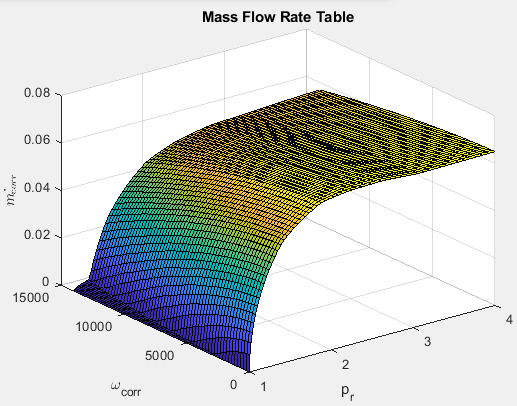#### Dependencies

To enable this parameter, select ```Fixed geometry``` for the Turbine type parameter.

Efficiency lookup table for fixed geometry, ${\eta }_{turbfx,tbl}$, as a function of corrected driveshaft speed, ωcorr, and pressure ratio, pr, dimensionless.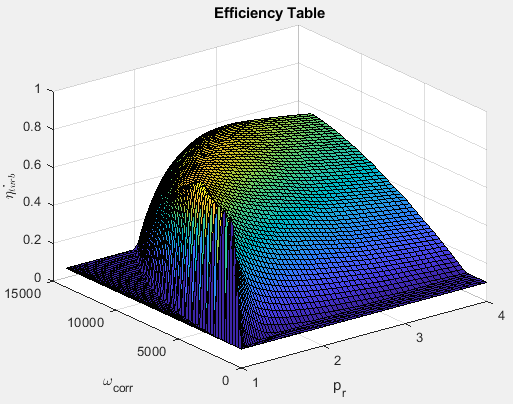#### Dependencies

To enable this parameter, select ```Fixed geometry``` for the Turbine type parameter.

Corrected drive shaft speed breakpoints for fixed geometry, ${\omega }_{corrfx,bpts1}$, in rad/s.

#### Dependencies

To enable this parameter, select ```Fixed geometry``` for the Turbine type parameter.

Pressure ratio breakpoints for fixed geometry, ${p}_{rfx,bpts2}$.

#### Dependencies

To enable this parameter, select ```Fixed geometry``` for the Turbine type parameter.

Corrected mass flow rate lookup table for variable geometry, ${\stackrel{˙}{m}}_{corrvr,tbl}$, as a function of corrected driveshaft speed, ωcorr, and pressure ratio, pr, in kg/s.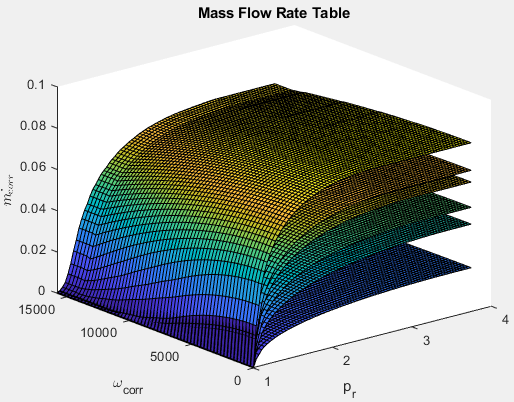#### Dependencies

To enable this parameter, select ```Variable geometry``` for the Turbine type parameter.

Efficiency lookup table for variable geometry, ${\eta }_{turbvr,tbl}$, as a function of corrected driveshaft speed, ωcorr, and pressure ratio, pr, dimensionless.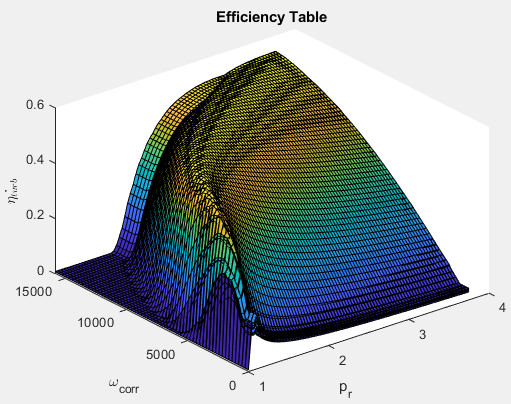#### Dependencies

To enable this parameter, select ```Variable geometry``` for the Turbine type parameter.

Corrected drive shaft speed breakpoints for variable geometry, ${\omega }_{corrvr,bpts1}$, in rad/s.

#### Dependencies

To enable this parameter, select ```Variable geometry``` for the Turbine type parameter.

Pressure ratio breakpoints for variable geometry.

#### Dependencies

To enable this parameter, select ```Variable geometry``` for the Turbine type parameter.

Rack position breakpoints for variable geometry, ${L}_{rack,bpts3}$.

#### Dependencies

To enable this parameter, select ```Variable geometry``` for the Turbine type parameter.

Performance map reference temperature, ${T}_{ref}$, in K.

Performance map reference pressure, ${P}_{ref}$, in Pa.

Wastegate

Area of fully opened wastegate valve, ${A}_{wgopen}$, in m^2.

#### Dependencies

To enable Wastegate flow area, A_wgopen, select the Include wastegate parameter.

#### Dependencies

Flow restriction linearization limit, ${p}_{lim,wg}$.

To enable Pressure ratio linearize limit, Plim_wg, select the Include wastegate parameter.

Properties

Ideal gas constant $R$, in J/(kg·K).

Specific heat at constant pressure, ${c}_{p}$, in J/(kg·K).

 Heywood, John B. Internal Combustion Engine Fundamentals. New York: McGraw-Hill, 1988.

 Eriksson, Lars and Lars Nielsen. Modeling and Control of Engines and Drivelines. Chichester, West Sussex, United Kingdom: John Wiley & Sons Ltd, 2014.